# Interpretations of Histogram

• Last Updated : 27 Jan, 2022

A Histogram is a variation of a bar chart in which data values are grouped together and put into different classes. This grouping enables you to see how frequently data in each class occur in the dataset.

The histogram graphically shows the following:

• Frequency of different data points in the dataset.
• Location of the center of data.
• Skewness/variance of dataset.
• Presence of outliers in the dataset.

The features provide a strong indication of the proper distributional model in the data. The probability plot or a goodness-of-fit test can be used to verify the distributional model.

The histogram contains the following axes:

• Vertical Axis: Frequency/count of each bin.
• Horizontal Axis: List of bins/categories.

#### Interpretations of Histogram:

• Normal Histogram: It is a classical bell-shaped histogram with most of the frequency counts focused in the middle with diminishing tails and there is symmetry with respect to the median. Since the normal distribution is most commonly observed in real-world scenarios, you are most likely to find these. In Normally distributed histogram mean is almost equal to median.
• Non-normal Short-tailed/ long-tailed histogram: In short-tailed distribution tail approaches 0 very fast, as we move from the median of data, In the long-tailed histogram, the tail approaches 0 slowly as we move far from the median. Here, we refer tail as the extreme regions in the histogram where most of the data is not-concentrated and this is on both sides of the peak.
• Bimodal Histogram: A mode of data represents the most common values in the histogram (i.e peak of the histogram. A bimodal histogram represents that there are two peaks in the histogram. The histogram can be used to test the unimodality of data. The bimodality (or for instance non-unimodality) in the dataset represents that there is something wrong with the process. Bimodal histogram many one or both of two characters: Bimodal normal distribution and symmetric distribution
• Skewed Left/Right Histogram: Skewed histogram are those where the one-side tail is quite clearly longer than the other-side tail. A right-skewed histogram means that the right-sided tail of the peak is more stretched than its left and vice-versa for the left-sided. In a left-skewed histogram, the mean is always lesser than the median, while in a right-skewed histogram mean is greater than the histogram.
• Uniform Histogram: In uniform histogram, each bin contains approximately the same number of counts (frequency). The example of uniform histogram is such as a die is rolled n (n>>30) number of times and record the frequency of different outcomes.
• Normal Distribution with an Outlier: This histogram is similar to normal histogram except it contains an outlier where the count/ probability of outcome is substantive. This is mostly due to some system errors in process, which led to faulty generation of products etc.

#### Implementation

• In this implementation, we will be using Numpy, Matplotlib and Seaborn plotting libraries. These libraries are pre-installed in colab, however for local environment, you can install these easily with pip install command.

## Python3

 `# Imports``import` `numpy as np``import` `matplotlib.pyplot as plt``import` `seaborn as sns` `# Normal histogram plot``data ``=` `np.random.normal(``10.0``, ``3``, ``500``)``sns.displot(data, kde``=` `True``, bins``=``10``, color``=``'black'``)` `# Left-skewed Histogram``wc_goals ``=``[``0``]``*` `19` `+` `[``1``]``*``49` `+` `[``2``]``*``60` `+` `[``3``] ``*``47` `+` `[``4``]``*``32` `+` `[``5``]``*` `18``+` `[``6``]``*``3` `+` `[``7``]``*``3` `+` `[``8``]``sns.displot(wc_goals, bins``=``8``, kde``=` `True``, alpha ``=``0.6``,color``=``'blue'``)` `# Right-skewed Histogram``wc_goals_conc ``=``    ``[``0``]``*` `19` `+` `[``-``1``]``*``49` `+` `[``-``2``]``*``60` `+` `[``-``3``] ``*``47` `+` `[``-``4``]``*``32` `+` `[``-``5``]``*` `18``+` `[``-``6``]``*``3` `+` `[``-``7``]``*``3` `+` `[``-``8``]``sns.displot(wc_goals_conc, kde ``=` `True``,bins``=``8``,  alpha``=``0.6``, color``=``'red'``)` `# Bi-modal histogram``N``=``400``mu_1, sigma_1 ``=` `80``, ``10``mu_2, sigma_2 ``=` `20``, ``10``# Generate two normal distributions of given mean sdand concatenate``X_1 ``=` `np.random.normal(mu, sigma, N)``X_2 ``=` `np.random.normal(mu2, sigma2, N)``X ``=` `np.concatenate([X1, X2])``sns.displot(X,bins``=``10``,kde``=``True` `, color``=``'green'``)` `# Uniform histogram (an example of die roll with N=600)``die_roll ``=`  `[``1``]``*``89` `+` `[``2``]``*``94` `+` `[``3``]``*``110` `+` `[``4``]``*``101` `+` `[``5``]``*``90` `+``[``6``]``*``116``sns.displot(die_roll, kde``=``True``, bins ``=``6``)` `# Normal distribution with an outlier``X_1 ``=` `np.random.normal(mu, sigma, N)``X_1 ``=``np.concatenate([X1, [``200``]``*``30``])``sns.displot(X_1, kde``=` `True``, bins``=``13``)`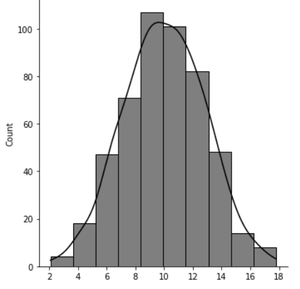Normal Histogram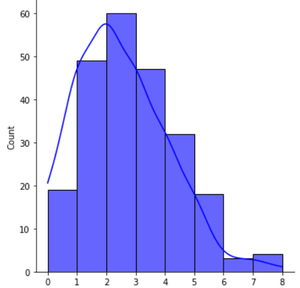Skewed Left Histogram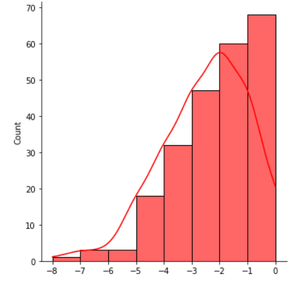Skewed-Right Histogram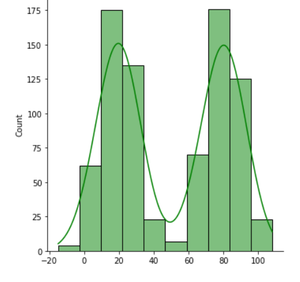Bi-modal Histogram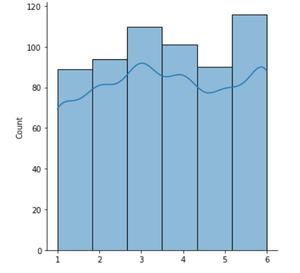Uniform Histogram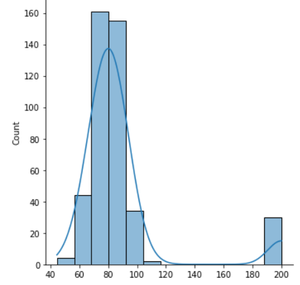Normal with an Outlier

My Personal Notes arrow_drop_up